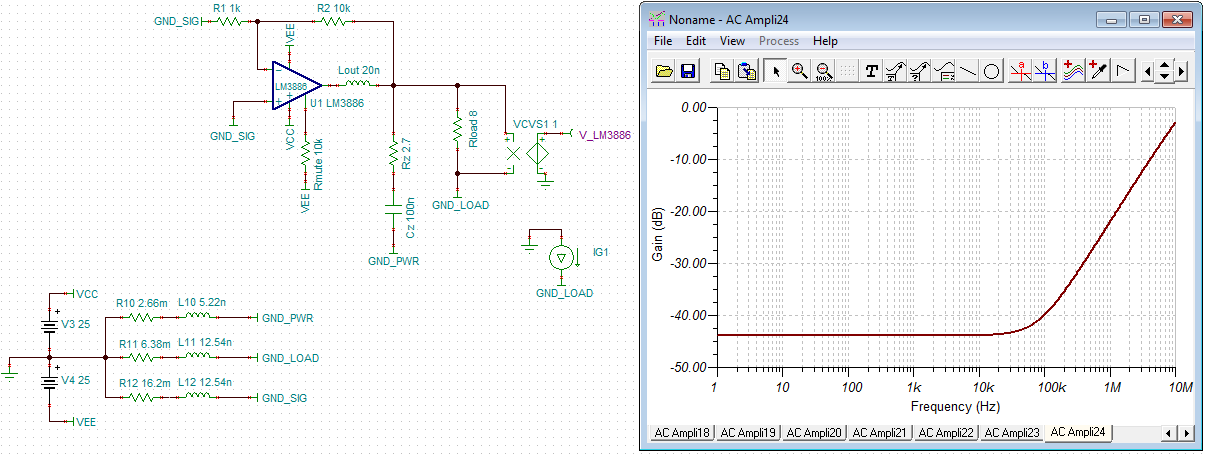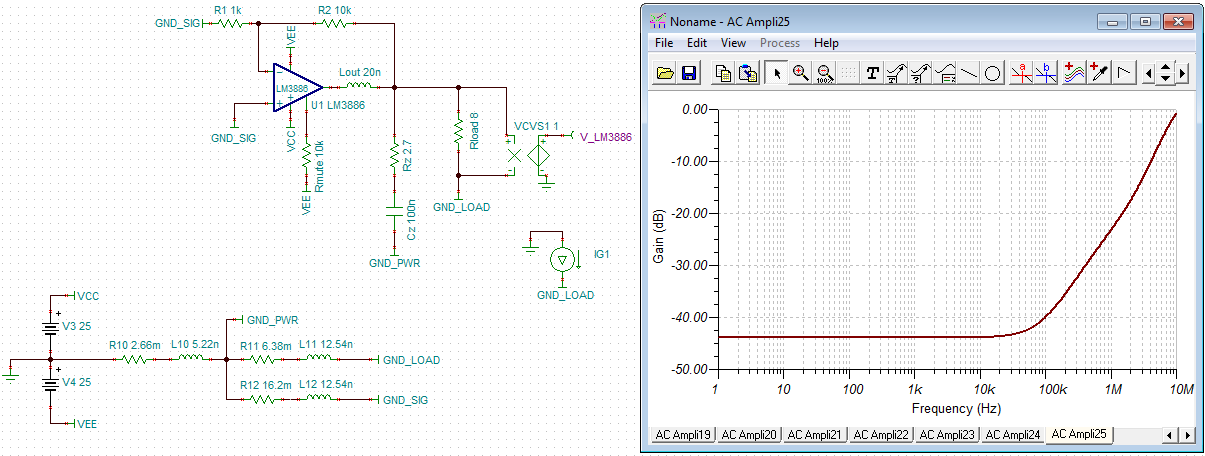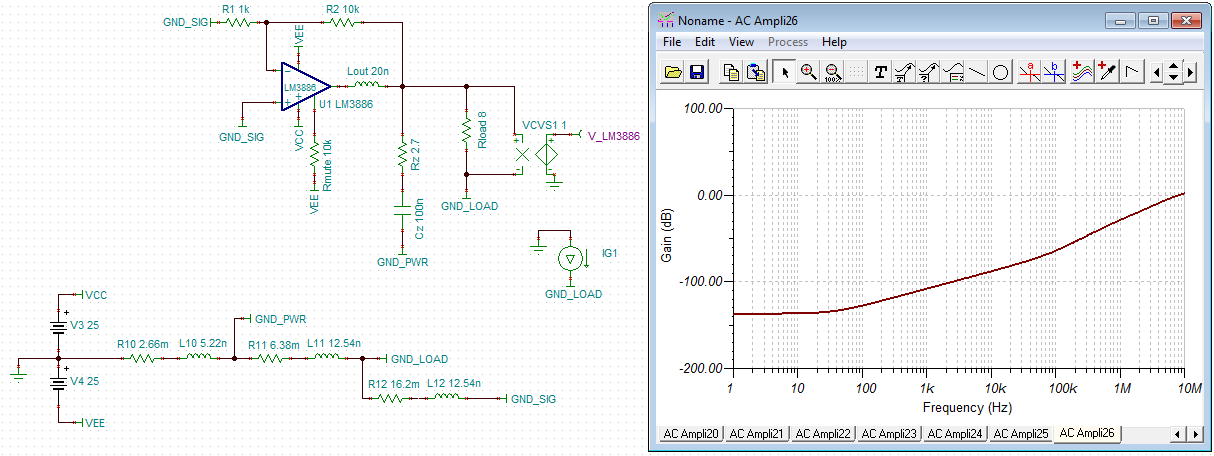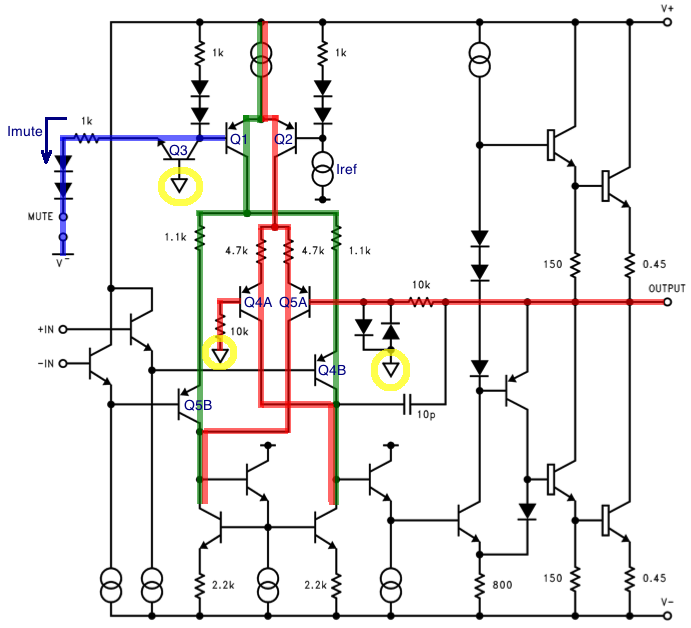# Grounding

As you have probably noticed, my knowledge base articles are free of advertising. Instead of distracting you with annoying ads, I kindly request your donation. If you find the contents of this page to be useful, please consider making a donation by clicking the Donate button below.Grounding: Section Outline

Casual reading of various audio fora quickly reveals that grounding is a perpetual cause of confusion. It seems most builders have a reasonably good grasp of how to route the VCC and VEE supplies, but the ground routing ranges from giant spider-like trace routes to a solid ground plane. This is a bit ironic, as one of the main functions of the ground routing is to provide a return path for the supply currents.

The Layout is the Circuit

The Grounding Conundrum starts at the data sheet with the figure on Page 21 of the LM3886 data sheet (reproduced below).The bottom schematic is touted as the holy grail and represents the Star Grounding scheme commonly used by DIY enthusiasts, confirmation bias prevails, and this is the topology implemented. However, in a mono block or small stereo amp configuration, this is not the most optimal layout. In fact, it is a rather poor layout.

The fundamental message that the layout has a big impact on circuit performance is still valid, however.

An Example of Star Grounding

The layout shown below is reasonably tight. The decoupling capacitors (C2, C4) are as close to the IC as possible and the main reservoir caps (C1, C3) are tucked in as well. This minimizes the supply inductance as discussed above. This layout is by no means optimal, but represents what a reasonably savvy DIYer could come up with. The ground trace (bottom layer - red) to the output connector is probably quite a bit shorter than it would be in real life as I have omitted the Thiele and Zobel networks.I operate with four "grounds":

1. GND: Simulator node 0, textbook ground. This is my reference point.
2. GND_PWR: The "ground star" at the local decoupling on the LM3886.
3. GND_SIG: Small signal ground. I.e. ground connection for the input connector and feedback connection.

The wide traces are 80 mil (2 mm) wide. The GND_SIG trace from R1 is 40 mil (1 mm) wide. By most people's standards, these are pretty fat traces. I used the Saturn PCB Toolkit to calculate the trace inductance and resistance. Using these parameters, I simulated the effects of the load return current on circuit performance.In the simulation, the inputs to the amplifier are grounded to GND_SIG and an AC current is injected into the GND_LOAD net. This represents the current returning from the speaker load. The return current results in an error voltage developing across the ground nets, part of which is amplified by the LM3886. The simulation uses a voltage controlled voltage source (VCVS) with a gain of 1 V/V to measure the resulting error voltage developed across the load. The resulting error voltage is plotted in dBV. Recall, the goal here is to have as little error voltage developed across the load as possible, as this error will degrade the THD performance of the amplifier. The simulation shows that for signals below 10 kHz, a 1 A return current will result in about 7.1 mV (-43 dBV) of differential voltage across the load. This is not very impressive performance.

Another common DIY solution is to use the center point between the local decoupling capacitors as the "star ground". That's not a bad idea, although, it does not improve the performance significantly as shown in the simulation below.Neither star grounding scheme works well. As the current returns from the load, it develops a voltage drop across the GND_LOAD impedance. This error voltage is subtracted from the desired voltage across the load, resulting in poor performance. The GND_SIG reference is taken at the ground star point, hence, any error voltage developed across the GND_LOAD net will remain unaffected by the amplifier's loop gain as it is outside the feedback loop.

It is possible to reduce the error voltage by optimizing the layout, minimizing the impedance of the GND_LOAD net. In the layout shown above, this would be trivial to do by swapping the connections to pins 1 and 2 of the output connector and by using a ground plane rather than a trace. The inductance per unit length of a PCB trace is reduced when the trace width is increased. A plane or pour is the widest trace possible, hence, a plane has the lowest possible inductance and lowest possible resistance. The proper use of ground planes and/or pours for high-current routes (supply pins, output pin) greatly improves circuit performance as the trace impedance is minimized. This will translate directly to improvements in the THD performance of the circuit.

There is more room for improvement, however. Significant improvement in circuit performance can be obtained by applying the loop gain of the amplifier to reduce this error voltage.

An Improved Grounding Scheme

From a feedback theory point-of-view, it seems apparent that if the voltage across the load is what we intend to control, the feedback voltage should be measured as close to the load as possible. This means that GND_SIG should connect to GND_LOAD. The resulting circuit is shown below along with the simulation result.Bingo! Now 1 A of returning load current will result in 100 nV (-140 dBV) of error voltage developed across the load for DC signals. Even at 10 kHz, the error voltage is only about 56 µV (-85 dBV) - a 40+ dB improvement over the star grounding scheme at 10 kHz and 95 dB improvement near DC. The only difference between the star ground and the improved grounding scheme is that the GND_SIG trace was routed to the output connector rather than to the star ground.

The layout is the circuit! I keep coming back to this statement as the mantra of precision circuit design.

The Mute Circuit

The final point of confusion is that pesky "GND" pin on the LM3886. Where should it connect? The short answer is: It doesn't really matter. Let's explore the equivalent circuit schematic for the LM3886 shown below.It may look a bit intimidating at first, but it can be broken down into sub-blocks pretty for ease of understanding. It turns out the LM3886 has two input stages. The input stage consisting of Q4B, Q5B, along with the two emitter followers connecting to +IN and -IN, is the one used during normal operation. The second input stage, Q4A, Q5A, is used when the output is muted. Switching between the two stages is done by Q1 and Q2 operating as an analog switch.

Consider the case where Imute = 0 and the amp is muted. Imute = 0 causes Q3 to turn off. Hence, the base of Q1 is pulled high through the two diodes and the 1 kΩ resistor. The base of Q2 is pulled low by Iref, hence, Q2 conducts the full input stage bias current. Q2 directs the current to the mute input stage, Q4A and Q5A. This current path is highlighted in red in the figure above. Q5A senses the voltage at the output of the amp. Q4A is biased to GND through a 10 kΩ resistor (matching the 10 kΩ on the base of Q5A). In this mode, the amplifier will be driven to present the voltage on the GND pin at the output of the amplifier.

As Imute is increased, Q3 will turn on. When Imute is sufficiently greater than Iref, Q1 and Q2 will switch, and all the input stage bias current now flows into the main input stage (Q4B, Q5B) along the path highlighted in green above. This enables normal operation of the amplifier.

By examination of the equivalent circuit schematic above, it becomes apparent that the voltage present at the GND pin of the IC is only relevant when the amplifier is muted as it is only used by the mute circuit. Unless you care tremendously about the sound quality when the amplifier is muted, exactly how the GND pin connects to ground does not matter. I would connect it to the ground by the decoupling caps as that's usually the closest available ground.

Section 4: Output Power